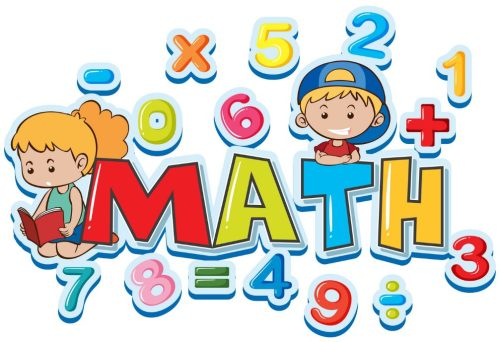(949) 864-9306

### Interesting MathIf the 26 letters of the English alphabets of: A, B, C, D, E…..X, Y, Z is converted to percentage respectively as listed below:

A=1% B =2% C=3% D=4% E=5% F=6% G=7% H=8% I=9% J=10% K=11% L=12% M=13% N=14% O=15% P=16% Q=17% R=18% S=19% T=20% U=21% V=22% W=23% X=24% Y=25% Z=26%

Then we would have the following interesting conclusions:

1. H+A+R+D+W+O+R+K = 8+1+18+4+23+15+18+11 = 98%

2. K+N+O+W+L+E+D+G+E = 11+14+15+23+12+5+4+7+5 = 96%

3. L+O+V+E = 12+5+22+5 = 54%

4. L+U+C+K = 12+21+3+11 = 47%

Then, all these above items which we value so much are not perfect, although they are important. Then what will let our lives become perfect?

5. Is it money? NO M+O+N+E+Y = 13+15+14+5+25 = 72%

6.Is it Leadership? NO L+E+A+D+E+R+S+H+I+P = 12+5+1+4+5+18+19+9+16 = 89%

7. Is it Sex? NO S+E+X = 19+24+5 = 48%

Then, what can make our lives 100% perfect?

8. It’s Attitude = 1+20+20+9+20+21+4+5 = 100%